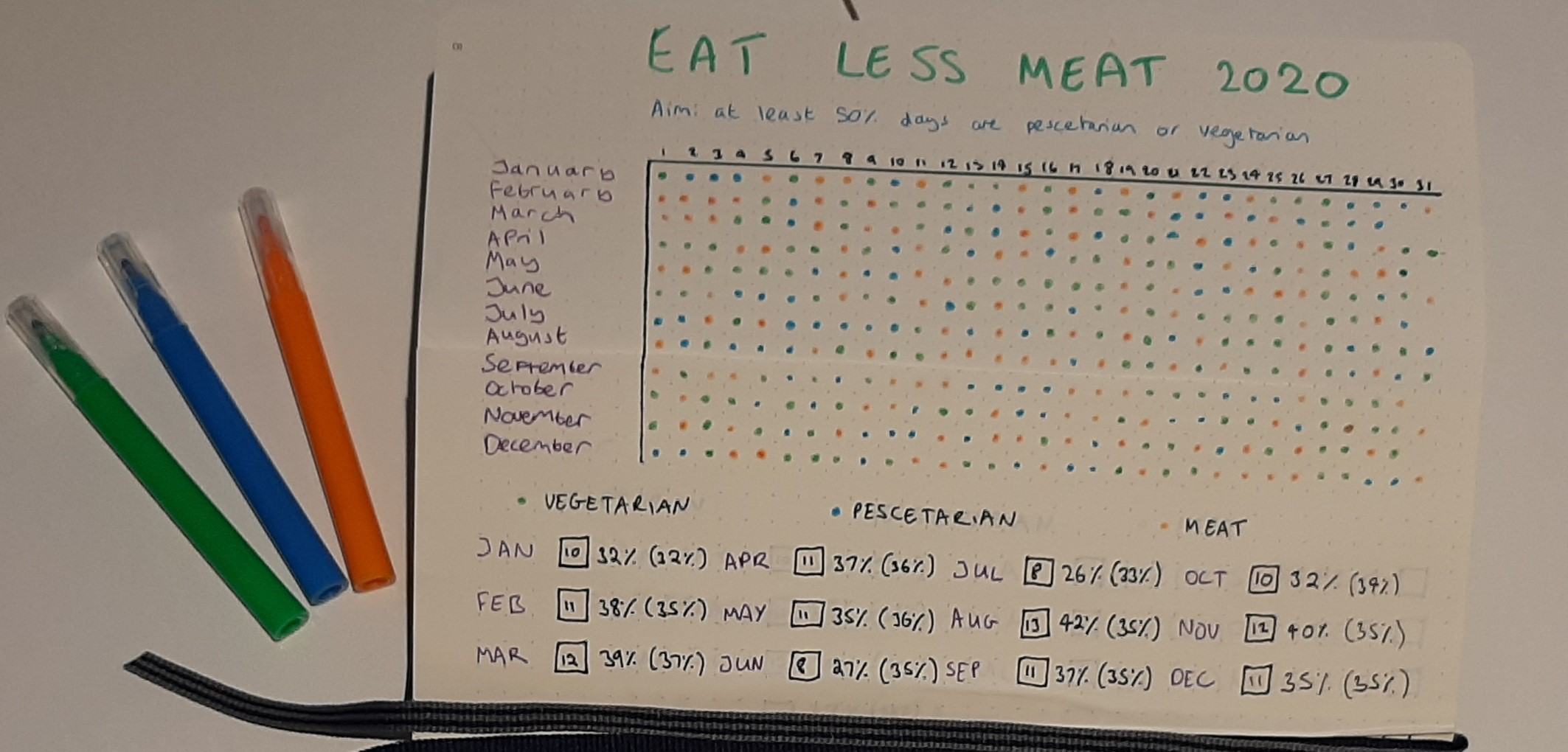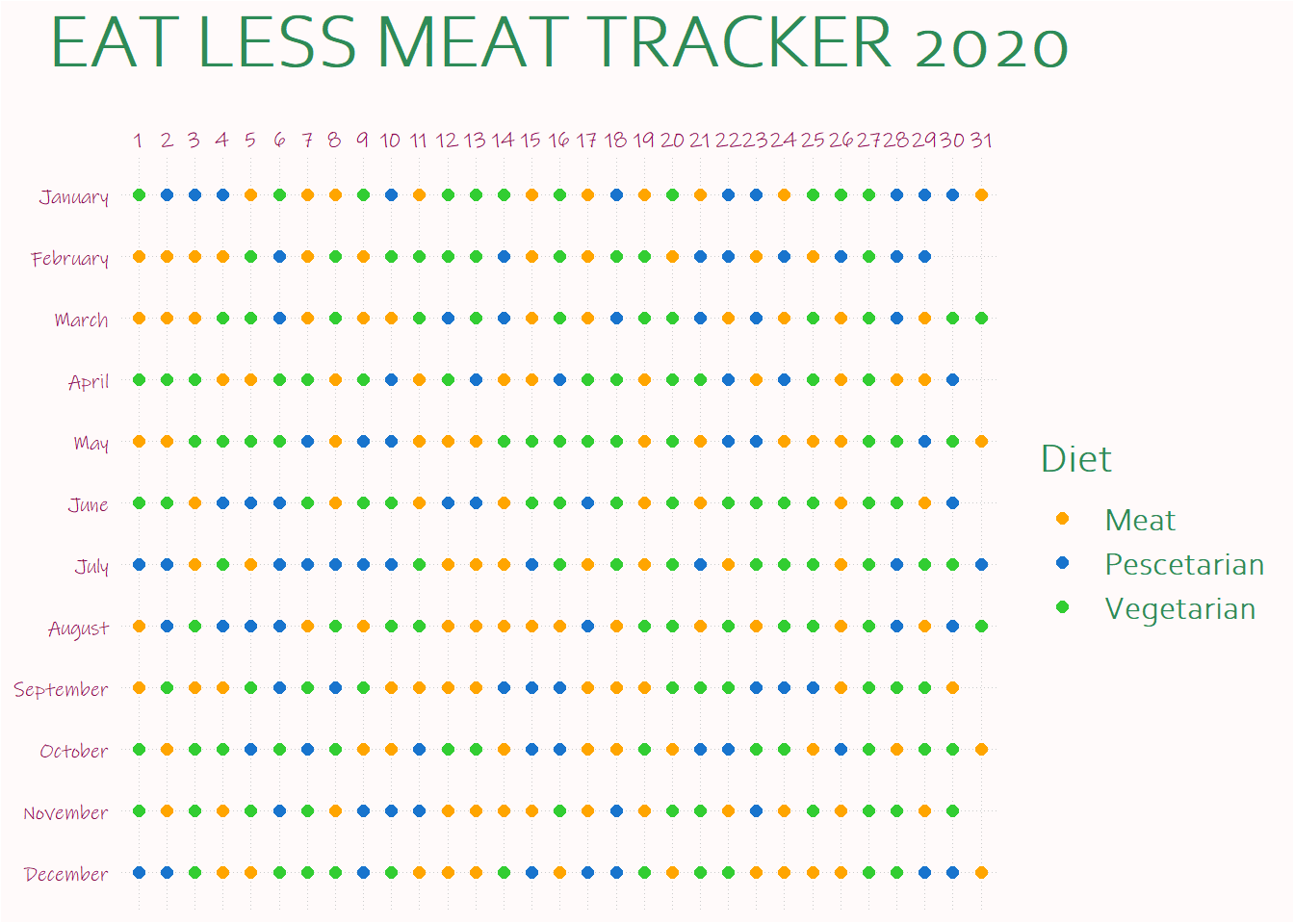# Page to plot

It’s the second day of January 2021, and a fitting time to be making resolutions. It’s also the time to look back at commitments made at the start of last year and check in on how they went. A lot of plans went awry in 2020, but staying home made it easier for me to stick to my resolution to eat less meat because I was doing a lot more meal planning. The result was I only ate meat on 35% of days, which was better than my original aspiration to cut it down to 50%.

This is super self-indulgent, and I apologise for that - but I did think it was quite a fun dataset to explore in more detail (for me, anyway). Because to keep track of what I was eating, I dedicated a page in my very underused bullet journal to the task, so I’ve got a log of whether each day was vegetarian, pescetarian or meat-eating. It looks like this:The first thing I wanted to do was replicate that chart using `ggplot2`. I started off by transcribing the data into a spreadhseet with the same dimensions and info.

``````# Load libraries and fonts
library(data.table)
library(ggplot2)

# Read in data
food``````
``````##         Month 1 2 3 4 5 6 7 8 9 10 11 12 13 14 15 16 17 18 19 20 21 22 23 24 25
##  1:   January V P P P M V M M V  P  M  V  V  V  M  V  M  P  M  V  M  P  P  M  V
##  2:  February M M M M V P M V M  V  V  V  V  P  M  V  M  V  V  M  P  P  M  P  M
##  3:     March M M M V V P M V M  M  V  P  V  P  M  V  M  P  V  V  P  M  P  M  V
##  4:     April V V V M M V V M V  P  M  V  P  M  M  P  V  V  M  V  V  P  M  P  V
##  5:       May M M V V V V P M P  P  M  M  M  V  V  V  V  V  M  V  M  P  P  M  M
##  6:      June V V M P P P V M V  V  M  P  P  M  V  V  P  V  M  V  M  V  V  V  V
##  7:      July P P M V M P P P P  P  V  M  M  M  P  V  M  V  M  V  P  M  V  V  V
##  8:    August M P V P P P M V M  V  V  M  M  M  M  M  P  M  V  V  M  V  M  V  V
##  9: September M V M M V P V P V  M  M  M  M  P  P  P  M  M  M  V  V  V  P  P  P
## 10:   October V M V V P V P V M  M  P  V  V  M  P  P  M  M  V  M  P  P  V  V  M
## 11:  November V M V M V P V M P  P  P  M  M  M  M  V  M  P  M  V  V  M  P  M  V
## 12:  December P P V M M V V V P  V  M  M  M  V  P  M  P  P  V  M  V  V  M  M  M
##     26 27 28 29 30 31
##  1:  V  V  P  P  P  M
##  2:  P  V  P  P
##  3:  M  V  P  M  V  V
##  4:  M  V  M  M  P
##  5:  M  V  V  P  V  M
##  6:  M  V  V  M  P
##  7:  M  V  P  V  V  P
##  8:  M  V  P  M  P  V
##  9:  M  V  V  V  M
## 10:  P  V  M  V  V  M
## 11:  M  V  V  M  V
## 12:  M  V  V  P  P  M``````

Then I adapted the data so it was a bit more user-friendly. I wanted one row per day, which will be useful for future analysis too.

``````# Transform data so one row per day
day_cols <- as.character(seq(1, 31))

food_by_day <- melt(food, id.vars = "Month", measure.vars = day_cols,
variable.name = "Day", value.name = "Diet")

food_by_day <- food_by_day[Diet != ""]
``````##       Month Day Diet
## 1:  January   1    V
## 2: February   1    M
## 3:    March   1    M
## 4:    April   1    V
## 5:      May   1    M
## 6:     June   1    V``````

And here’s my attempt to roughly replicate the page from my journal.

``````# Months in correct order in factor
food_by_day[, Month := factor(Month, levels = unique(Month))]

# Colours for plot
diet_cols <- c("orange", "dodgerblue3", "limegreen")

# Plot
ggplot(food_by_day, aes(Day, Month, colour = Diet)) +
geom_point(size = 2) +
theme(
plot.background = element_rect(fill = "snow1"),
legend.background = element_rect(fill = "snow1"),
panel.background = element_rect(fill = "snow1"),
text = element_text(colour = "seagreen4", family = "Corbel Light",
size = 16, face = "bold"),
axis.text = element_text(colour = "deeppink4",
family = "Ink Free", size = 8, face = "plain"),
axis.title = element_blank(),
panel.grid.major = element_line(colour = "gray80",
size = 0.1,
linetype = "dotted"),
plot.title = element_text(size = 30, hjust = 0.5,
margin=margin(0,0,15,0)),
axis.ticks = element_blank(),
legend.key = element_rect(fill = "snow1")
) +
scale_y_discrete(limits = rev(levels(food_by_day\$Month))) +
scale_x_discrete(position = "top") +
scale_color_manual(values = diet_cols, labels = c("Meat",
"Pescetarian",
"Vegetarian")) +
labs(title = "EAT LESS MEAT TRACKER 2020")``````Next, I’m going to do some data exploration and try to find out if there are any interesting patterns - particularly if they help me do better this year.